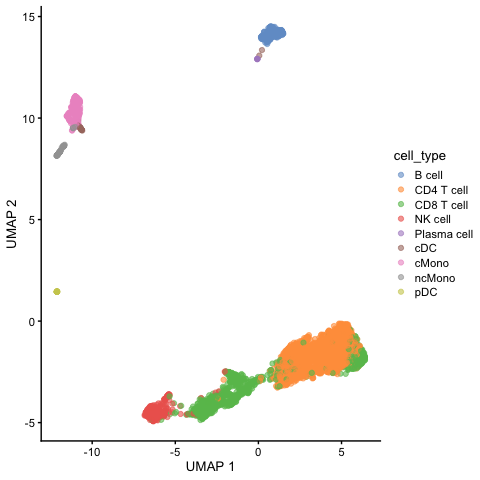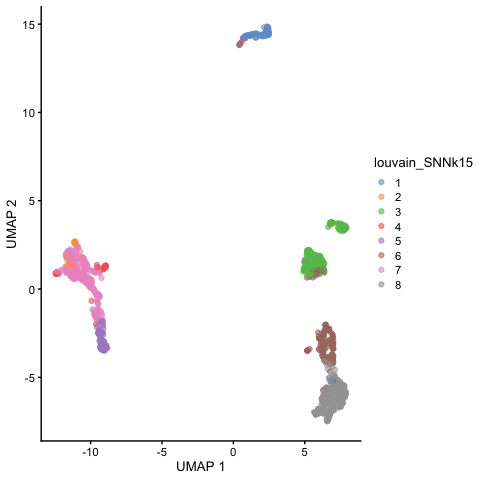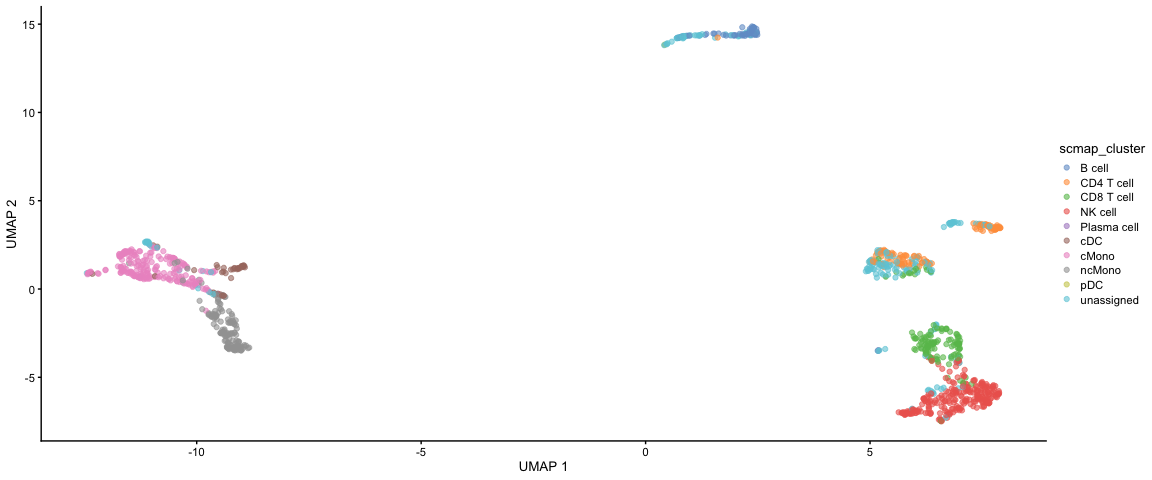## Celltype prediction

Celltype prediction can either be performed on indiviudal cells where each cell gets a predicted celltype label, or on the level of clusters. All methods are based on similarity to other datasets, single cell or sorted bulk RNAseq, or uses know marker genes for each celltype.

We will select one sample from the Covid data, `ctrl_13` and predict celltype by cell on that sample.

Some methods will predict a celltype to each cell based on what it is most similar to even if the celltype of that cell is not included in the reference. Other methods include an uncertainty so that cells with low similarity scores will be unclassified. There are multiple different methods to predict celltypes, here we will just cover a few of those.

Here we will use a reference PBMC dataset from the `scPred` package which is provided as a Seurat object with counts. And we will test classification based on the `scPred` and `scMap` methods. Finally we will use gene set enrichment predict celltype based on the DEGs of each cluster.

## Covid-19 data

First, lets load required libraries and the saved object from the clustering step. Subset for one patient.

``````suppressPackageStartupMessages({
library(scater)
library(scran)
library(dplyr)
library(cowplot)
library(ggplot2)
library(pheatmap)
library(rafalib)
library(scPred)
library(scmap)
})``````
``````# load the data and select 'ctrl_13` sample
ctrl.sce <- alldata[, alldata@colData\$sample == "ctrl.13"]

# remove all old dimensionality reductions as they will mess up the analysis
# further down
reducedDims(ctrl.sce) <- NULL``````

## Reference data

Then, load the reference dataset with annotated labels. Also, run all steps of the normal analysis pipeline with normalizaiton, variable gene selection, scaling and dimensionality reduction.

``````reference <- scPred::pbmc_1

reference``````
``````## An object of class Seurat
## 32838 features across 3500 samples within 1 assay
## Active assay: RNA (32838 features, 0 variable features)``````

Convert to a SCE object.

``ref.sce = Seurat::as.SingleCellExperiment(reference)``

## Rerun analysis pipeline

Run normalization, feature selection and dimensionality reduction

``````# Normalize
ref.sce <- computeSumFactors(ref.sce)
ref.sce <- logNormCounts(ref.sce)

# Variable genes
var.out <- modelGeneVar(ref.sce, method = "loess")
hvg.ref <- getTopHVGs(var.out, n = 1000)

# Dim reduction
ref.sce <- runPCA(ref.sce, exprs_values = "logcounts", scale = T, ncomponents = 30,
subset_row = hvg.ref)
ref.sce <- runUMAP(ref.sce, dimred = "PCA")``````
``plotReducedDim(ref.sce, dimred = "UMAP", colour_by = "cell_type")``Run all steps of the analysis for the ctrl sample as well. Use the clustering from the integration lab with resolution 0.3.

``````# Normalize
ctrl.sce <- computeSumFactors(ctrl.sce)
ctrl.sce <- logNormCounts(ctrl.sce)

# Variable genes
var.out <- modelGeneVar(ctrl.sce, method = "loess")
hvg.ctrl <- getTopHVGs(var.out, n = 1000)

# Dim reduction
ctrl.sce <- runPCA(ctrl.sce, exprs_values = "logcounts", scale = T, ncomponents = 30,
subset_row = hvg.ctrl)
ctrl.sce <- runUMAP(ctrl.sce, dimred = "PCA")``````
``plotReducedDim(ctrl.sce, dimred = "UMAP", colour_by = "louvain_SNNk15")``# scMap

The scMap package is one method for projecting cells from a scRNA-seq experiment on to the cell-types or individual cells identified in a different experiment. It can be run on different levels, either projecting by cluster or by single cell, here we will try out both.

## scMap cluster

For scmap cell type labels must be stored in the `cell_type1` column of the `colData` slots, and gene ids that are consistent across both datasets must be stored in the `feature_symbol` column of the `rowData` slots. Then we can select variable features in both datasets.

``````# add in slot cell_type1
ref.sce@colData\$cell_type1 = ref.sce@colData\$cell_type
# create a rowData slot with feature_symbol
rd = data.frame(feature_symbol = rownames(ref.sce))
rownames(rd) = rownames(ref.sce)
rowData(ref.sce) = rd

# same for the ctrl dataset create a rowData slot with feature_symbol
rd = data.frame(feature_symbol = rownames(ctrl.sce))
rownames(rd) = rownames(ctrl.sce)
rowData(ctrl.sce) = rd

# select features
counts(ctrl.sce) <- as.matrix(counts(ctrl.sce))
logcounts(ctrl.sce) <- as.matrix(logcounts(ctrl.sce))
ctrl.sce <- selectFeatures(ctrl.sce, suppress_plot = TRUE)

counts(ref.sce) <- as.matrix(counts(ref.sce))
logcounts(ref.sce) <- as.matrix(logcounts(ref.sce))
ref.sce <- selectFeatures(ref.sce, suppress_plot = TRUE)``````

Then we need to index the reference dataset by cluster, default is the clusters in `cell_type1`.

``ref.sce <- indexCluster(ref.sce)``

Now we project the Covid-19 dataset onto that index.

``````project_cluster <- scmapCluster(projection = ctrl.sce, index_list = list(ref = metadata(ref.sce)\$scmap_cluster_index))

# projected labels
table(project_cluster\$scmap_cluster_labs)``````
``````##
##      B cell  CD4 T cell  CD8 T cell     NK cell Plasma cell         cDC
##          66         108         133         256           3          38
##       cMono      ncMono         pDC  unassigned
##         217         144           2         208``````

``````# add in predictions
ctrl.sce@colData\$scmap_cluster <- project_cluster\$scmap_cluster_labs

plotReducedDim(ctrl.sce, dimred = "UMAP", colour_by = "scmap_cluster")``````## scMap cell

We can instead index the refernce data based on each single cell and project our data onto the closest neighbor in that dataset.

``ref.sce <- indexCell(ref.sce)``

Again we need to index the reference dataset.

``project_cell <- scmapCell(projection = ctrl.sce, index_list = list(ref = metadata(ref.sce)\$scmap_cell_index))``

We now get a table with index for the 5 nearest neigbors in the reference dataset for each cell in our dataset. We will select the celltype of the closest neighbor and assign it to the data.

``````cell_type_pred <- colData(ref.sce)\$cell_type1[project_cell\$ref[][1, ]]

table(cell_type_pred)``````
``````## cell_type_pred
##      B cell  CD4 T cell  CD8 T cell     NK cell Plasma cell         cDC
##          98         172         316         161           2          36
##       cMono      ncMono         pDC
##         209         179           2``````

``````# add in predictions